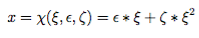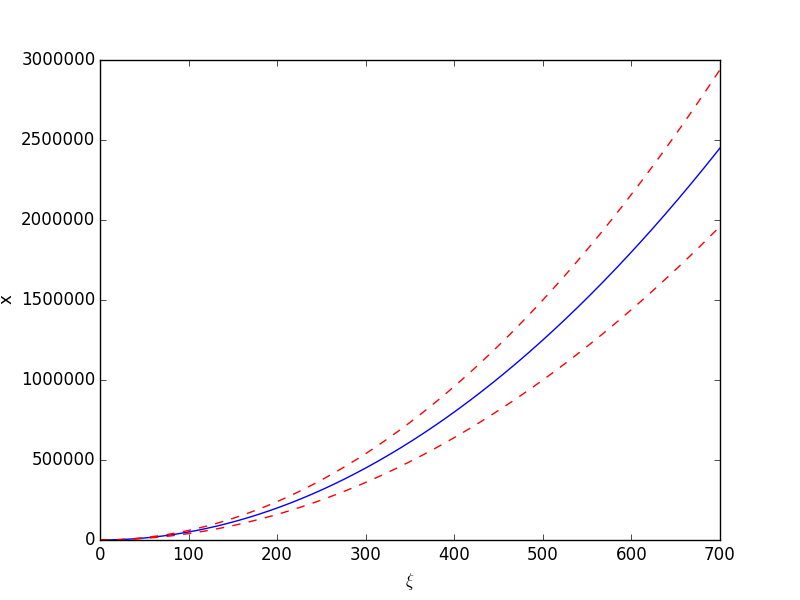# Chaofeng Wang

### Computational Mechanics, Deep Learning, Uncertainty Quantification, Natural Hazards5 years ago in Research, blog · 1 MIN READ

# Polynomial chaos expansion

Polynomial chaos expansion (PCE) received much attention in applied sciences in recent years. As a novel method to determine the evolution of uncertainty, which origin from the probabilistic uncertainty in the system parameters, in a complex system, PCE has been found to be an attractive and promising numerical method to uncertain quantification.

The concept of PCE is to use a series of weighted orthogonal polynomials to approximate a function.where φi is the mode function and βi is the model strength. The expansion in the above is a PCE. The strength is calculated aswhere the notation <, > means the inner product of two functions with respect to the prob- ability density function of the parameter Ξ. The selection of polynomials associated to different distributions of Ξ:An example application:where ε follows an uniform distribution on [-1, 1] and ζ follows a normal distribution N(5,1). The expected value of x and its standard deviation is···#### Chaofeng Wang

© 2023 Chaofeng Wang · Built on Canvas / Laravel